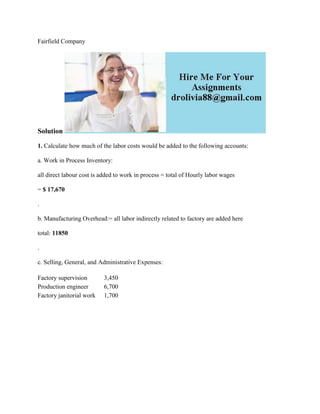Seu SlideShare está sendo baixado. ×

# Fairfield CompanySolution1- Calculate how much of the labor costs woul.docx

Anúncio
Anúncio
Anúncio
Anúncio
Anúncio
Anúncio
Anúncio
Anúncio
Anúncio
Anúncio
AnúncioCarregando em…3
×

1 de 2 Anúncio

# Fairfield CompanySolution1- Calculate how much of the labor costs woul.docx

Fairfield Company
Solution
1. Calculate how much of the labor costs would be added to the following accounts:
a. Work in Process Inventory:
all direct labour cost is added to work in process = total of Hourly labor wages
= \$ 17,670
.
b. Manufacturing Overhead:= all labor indirectly related to factory are added here
total: 11850
.
c. Selling, General, and Administrative Expenses:

.

Fairfield Company
Solution
1. Calculate how much of the labor costs would be added to the following accounts:
a. Work in Process Inventory:
all direct labour cost is added to work in process = total of Hourly labor wages
= \$ 17,670
.
b. Manufacturing Overhead:= all labor indirectly related to factory are added here
total: 11850
.
c. Selling, General, and Administrative Expenses:

.

Anúncio
Anúncio

### Fairfield CompanySolution1- Calculate how much of the labor costs woul.docx

1. 1. Fairfield Company Solution 1. Calculate how much of the labor costs would be added to the following accounts: a. Work in Process Inventory: all direct labour cost is added to work in process = total of Hourly labor wages = \$ 17,670 . b. Manufacturing Overhead:= all labor indirectly related to factory are added here total: 11850 . c. Selling, General, and Administrative Expenses: Factory supervision 3,450 Production engineer 6,700 Factory janitorial work 1,700